RRB NTPC Mock Test - 13 (English)

# RRB NTPC Mock Test - 13 (English)

Test Description

## 100 Questions MCQ Test RRB NTPC Mock Tests Series | RRB NTPC Mock Test - 13 (English)

RRB NTPC Mock Test - 13 (English) for Railways 2022 is part of RRB NTPC Mock Tests Series preparation. The RRB NTPC Mock Test - 13 (English) questions and answers have been prepared according to the Railways exam syllabus.The RRB NTPC Mock Test - 13 (English) MCQs are made for Railways 2022 Exam. Find important definitions, questions, notes, meanings, examples, exercises, MCQs and online tests for RRB NTPC Mock Test - 13 (English) below.
Solutions of RRB NTPC Mock Test - 13 (English) questions in English are available as part of our RRB NTPC Mock Tests Series for Railways & RRB NTPC Mock Test - 13 (English) solutions in Hindi for RRB NTPC Mock Tests Series course. Download more important topics, notes, lectures and mock test series for Railways Exam by signing up for free. Attempt RRB NTPC Mock Test - 13 (English) | 100 questions in 90 minutes | Mock test for Railways preparation | Free important questions MCQ to study RRB NTPC Mock Tests Series for Railways Exam | Download free PDF with solutions
 1 Crore+ students have signed up on EduRev. Have you?
RRB NTPC Mock Test - 13 (English) - Question 1

### How many pairs of Spinal nerves are found in humans?

Detailed Solution for RRB NTPC Mock Test - 13 (English) - Question 1
• 31 pairs of Spinal nerves are found in humans.
• Out of the 31 pairs, 8 cervical12 thoracic5 lumbar5 sacral and 1 coccygeal.
• 10 pairs of Spinal nerves are found in frogs.
• 37 pairs of Spinal nerves are found in rabbits.
RRB NTPC Mock Test - 13 (English) - Question 2

### A sum amounts to Rs. 2420 in 2 years when invested at compound interest. If principal is Rs. 2000, then what is the rate of interest (in %)?

Detailed Solution for RRB NTPC Mock Test - 13 (English) - Question 2

Principal Amount = Rs. 2000

Amount = Rs. 2420

Number of years = 2

Amount = P(1 + r/100)t, where P is the principal, r is the rate of interest and t is the time period

2420 = 2000 (1 + r/100)2

1.21 = 1.0201r2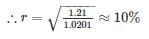RRB NTPC Mock Test - 13 (English) - Question 3

### Which among the following place is known as the Pearl of the Danube?

Detailed Solution for RRB NTPC Mock Test - 13 (English) - Question 3

Budapest is known as the Pearl of the Danube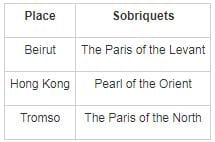RRB NTPC Mock Test - 13 (English) - Question 4

A truck covers a distance of 1925 km in 35 hours. What is the average speed of the truck?

Detailed Solution for RRB NTPC Mock Test - 13 (English) - Question 4

Let, the average speed of truck = x km/hr

According to problem,

⇒ 35x = 1925

⇒ x = 55

∴ average speed of the truck = 55 km/hr

RRB NTPC Mock Test - 13 (English) - Question 5

What is the name of the first Indian satellite?

Detailed Solution for RRB NTPC Mock Test - 13 (English) - Question 5
• Aryabhatta was the first satellite launched by India.
• It was launched on April 19, 1975, from Kapustin Yar.
• Rohini became the first satellite to be placed in orbit by an Indian-made launch vehicle.
• Sputnik 1 was the world’s first artificial satellite launched by the Soviet Union.
RRB NTPC Mock Test - 13 (English) - Question 6

If income of R is 30% more than the income of Q and the income of Q is 20% more than the income of P, then the income of R is how much percent more than the income of P?

Detailed Solution for RRB NTPC Mock Test - 13 (English) - Question 6

Let income of P be x

The income of Q is 20% more than P

So income of Q = x + 20x/100 = 1.2x

The income of R is 30% more than Q.

So income of R = 1.2x + (30 × 1.2x)/100 = 1.56x

% difference in incomes of R and P = [(Income of R - Income of P)/Income of P] × 100%

= [(1.56x - x)/x] × 100% = 56%

RRB NTPC Mock Test - 13 (English) - Question 7

The Telecom Regulatory Authority of India was set up in the year ________.

Detailed Solution for RRB NTPC Mock Test - 13 (English) - Question 7

● The Telecom Regulatory Authority of India (TRAI) was established with effect from 20th February 1997 by an Act of Parliament, called the Telecom Regulatory Authority of India Act, 1997.

● It was established to regulate telecom services, including fixation/revision of tariffs for telecom services.

● One of the main objectives of TRAI is to provide a fair and transparent policy environment which promotes a level playing field and facilitates fair competition.

● Chairman: Ram Sewak Sharma

RRB NTPC Mock Test - 13 (English) - Question 8

If sum of the roots of a quadratic equations is 1 and product of the roots is – 20. Find the quadratic equation.

Detailed Solution for RRB NTPC Mock Test - 13 (English) - Question 8

Sum of roots = 1

Product of root = – 20

Quadratic equation ⇒ X2 – (Sum of root) X + (Product of root) = 0

⇒ X2 – (1) X + (– 20) = 0

⇒ X2 – X – 20 = 0

Hence, required quadratic equation will be X2 – X – 20 = 0

RRB NTPC Mock Test - 13 (English) - Question 9

If Sin A + Cos A = 0.5, find the value of Sin A.Cos A = ?

Detailed Solution for RRB NTPC Mock Test - 13 (English) - Question 9

(Sin A + Cos A)² = Sin² A + Cos² A + 2 Sin A Cos A

⇒ (0.5)² = 1 + 2 Sin A Cos A

⇒ 0.25 – 1 = 2 Sin A Cos A

⇒ 2 Sin A Cos A = -0.75

∴ Sin A Cos A = ​-0.375

RRB NTPC Mock Test - 13 (English) - Question 10

Peter Tabichi won the Global Teacher Prize for 2019 in Dubai. He is from which of the following country?

Detailed Solution for RRB NTPC Mock Test - 13 (English) - Question 10

● A Maths and Physics teacher, Peter Tabichi from a secondary school in a remote village in Kenya’s Rift Valley has won the \$1 million Global Teacher Prize for 2019.

● He gives away 80 per cent of his income to help the poor in the remote village of Pwani where almost a third of the children are orphans or have only one parent.

● He received the prize at a ceremony Saturday in Dubai hosted by Hollywood star Hugh Jackman.

● He has become the first ever African male to have won the award.

RRB NTPC Mock Test - 13 (English) - Question 11

Sameer and Raman met at airport. Sameer was with a lady. He introduced that lady to Raman saying “her father is the brother of my mother’s mother – in - law” How is the woman related to Sameer?

Detailed Solution for RRB NTPC Mock Test - 13 (English) - Question 11

Sameer’s mother’s mother in law → Sameer’s grandmother.

That lady’s father is the brother of Sameer’s grandmother.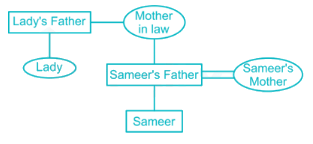So, the woman is Sameer’s aunt.

RRB NTPC Mock Test - 13 (English) - Question 12

In which railway region did the automatic signal system start?

Detailed Solution for RRB NTPC Mock Test - 13 (English) - Question 12
• Automatic signal system was started in the Central Railway.
• Its headquarters is in Mumbai at Chhatrapati Shivaji Maharaj Terminus.
• It was founded on 5 November 1951, Mumbai.
• There are total of 18 railway zones in India.
RRB NTPC Mock Test - 13 (English) - Question 13

A number is increased first by 20% and then it is again increased by 15%. What is the percentage change in the number?

Detailed Solution for RRB NTPC Mock Test - 13 (English) - Question 13

Let, the number = x

After 20% increase, number = x + x × 20/100 = 1.2x

After 15% increase, number = 1.2x + 1.2x × 15/100 = 1.38x

∴ Increase = 1.38x - x = 0.38x

∴ Percentage increase,

⇒ 0.38x/x × 100%

⇒ 38%

RRB NTPC Mock Test - 13 (English) - Question 14

U, V and W together can complete a work in 15 days. U alone can complete the work in 60 days. In how many days V and W together can complete the same work?

Detailed Solution for RRB NTPC Mock Test - 13 (English) - Question 14

Given,

(U + V + W) can complete a work in 15 days.

⇒ (U + V + W)’s one day work = 1/15

U alone can complete the work in 60 days.

⇒ U’s one day work = 1/60

⇒ (V + W)’s one day work = (1/15 - 1/60) = (4 - 1)/60 = 1/20

V + W can complete the same work in 20 days.

RRB NTPC Mock Test - 13 (English) - Question 15

To which of the following dynasty, Shivaskandavarman belongs?

Detailed Solution for RRB NTPC Mock Test - 13 (English) - Question 15

● Shivaskandavarman is known as the founder of the Pallava Dynasty.

● The Pallava Dynasty is said to have emerged in South India when the Satavahana dynasty was on the decline.

● During their reign, Pallavas made Kanchi as their capital.

● Pallavas became a major power during the reign of Mahendravarman I and Narasimhavarman I.

RRB NTPC Mock Test - 13 (English) - Question 16

Which answer figure will complete the pattern in the question figure?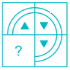Detailed Solution for RRB NTPC Mock Test - 13 (English) - Question 16Thus image at option 1 completes the given pattern.

RRB NTPC Mock Test - 13 (English) - Question 17

Which of the following jumble word is not the name of a major book of any country?

Detailed Solution for RRB NTPC Mock Test - 13 (English) - Question 17

1) UELBOOKB = BLUEBOOK (An official report of the British Government)

2) REENGOOKB = GREENBOOK (An official publication of Iran and Italy)

3) ERDOOKB = REDBOOK (Not an official book)

4) ITEWHOOKB = WHITEBOOK (An official publication of China)

Hence option ERDOOKB is the correct answer.

RRB NTPC Mock Test - 13 (English) - Question 18

Directions: What will come in place of the question mark (?) in the following questions?
4015 × 73 - 21817 = ? × 112 + 126

Detailed Solution for RRB NTPC Mock Test - 13 (English) - Question 18

Follow BODMAS rule to solve this question, as per the order given below,

Step-1- Parts of an equation enclosed in 'Brackets' must be solved first, and in the bracket, the BODMAS rule must be followed,

Step-2- Any mathematical 'Of' or 'Exponent' must be solved next,

Step-3-Next, the parts of the equation that contain 'Division' and 'Multiplication' are calculated,

Step-4-Last but not least, the parts of the equation that ​contain 'Addition' and 'Subtraction' should be calculated.

4015 × 73 - 21817 = ? × 112 + 126

⇒ 293095 - 21817 = ? × 112 + 126

⇒ 271278 = ? × 112 + 126

⇒ ? × 112 = 271278 - 126

⇒ ? = 271152/112

∴ ? = 2421

RRB NTPC Mock Test - 13 (English) - Question 19

Which of the following states is the largest producer of bamboo in India?

Detailed Solution for RRB NTPC Mock Test - 13 (English) - Question 19

Assam is the largest bamboo producing state in India.

India is the second largest producer of bamboo behind China.

Bamboo is used for multiple purposes like in the construction of buildings and in the making of furniture and various devices.

Assam also has a Bamboo Technology Park worth Rs. 62.28 crore build in 2017 at the Chaygaon in Kamrup district.

RRB NTPC Mock Test - 13 (English) - Question 20

The product of two positive numbers is 2500. If one number is four times the other, then what will be the sum of the two numbers?

Detailed Solution for RRB NTPC Mock Test - 13 (English) - Question 20

Let the two numbers be x and y.

Given,

xy = 2500

And x is four times y.

⇒ x = 4y      ----(1)

Putting the value of x in (1), we get

⇒ 4y2 = 2500

⇒ y2 = 625

⇒ y = 25

The numbers are x = 4 × y = 100 and y = 25

Sum of two numbers = 100 + 25 = 125

Previous

RRB NTPC Mock Test - 13 (English) - Question 21

What least number must be added to 41116, so that the sum is completely divisible by 8?

Detailed Solution for RRB NTPC Mock Test - 13 (English) - Question 21

Divisibility rule of 8: If the last three digits of a whole number are divisible by 8, then the entire number is divisible by 8.

The last three digits of the given number are 116

116 = 14 x 8 + 4

As the remainder is 4, the least number to be added to make it divisible by 8 is 8 - 4 = 4

The least number that should be added to 116 to make it divisible by 8 is 4.

RRB NTPC Mock Test - 13 (English) - Question 22

Who among the following invented Barometer?

Detailed Solution for RRB NTPC Mock Test - 13 (English) - Question 22
• Evangelista Torricelli invented Barometer.
• It was invented in 1644 in Italy.
• It is used for measuring atmospheric pressure, used especially in forecasting the weather and determining altitude.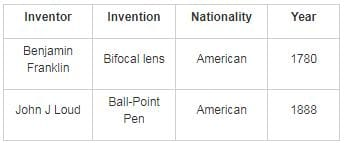RRB NTPC Mock Test - 13 (English) - Question 23

Kamal remembers his birthday is before 24th April. His mother remembers that his birthday is after 22nd April. On which date of April, is Kamal's birthday?

Detailed Solution for RRB NTPC Mock Test - 13 (English) - Question 23

Kamal remembers his birthday is before 24th April (i.e. on 23rd, 22nd, 21st… etc.) while his mother remembers that his birthday is after 22nd April (i.e. on 23rd, 24th, 25th… etc.). Combining both the statements we get only one possible date which is 23rd April.

Hence, 23 is the correct alternative.

RRB NTPC Mock Test - 13 (English) - Question 24

Who is known as the Father of English Poetry?

Detailed Solution for RRB NTPC Mock Test - 13 (English) - Question 24

Geoffrey Chaucer is known as the "Father of English Poetry".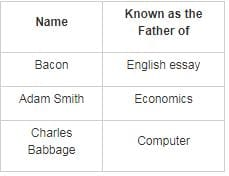RRB NTPC Mock Test - 13 (English) - Question 25

In the following question below are given some statements followed by some conclusions. Taking the given statements to be true even if they seem to be at variance from commonly known facts, read all the conclusions and then decide which of the given conclusion logically follows the given statements.

Statements: I. Some tables are chairs. II. All chairs are cups.

Conclusions: I. All tables are cups. II. Some tables are cups

Detailed Solution for RRB NTPC Mock Test - 13 (English) - Question 25

Consider the following least possible Venn diagram,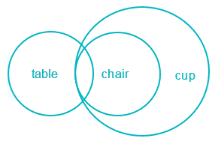Conclusions:

I. All tables are cups → False (It is possible but not definite).

II. Some tables are cups → True (It is definitely true as some tables are chairs and all chairs are cups).

Hence, only conclusion II follows.

RRB NTPC Mock Test - 13 (English) - Question 26

Choose the odd number among the numbers given below.

Detailed Solution for RRB NTPC Mock Test - 13 (English) - Question 26

3375, 2744 and 8 are cubes of 15, 14 and 2 respectively, but 980 is not the cube of 1.

Hence, 980 is the odd one out.

RRB NTPC Mock Test - 13 (English) - Question 27

The second Carnatic war was fought between _______ and ________.

Detailed Solution for RRB NTPC Mock Test - 13 (English) - Question 27
• All of the Carnatic wars were military conflicts between French East India and the British East India Company in the 18th century.
• The French wanted to reduce the growing influence of the English in the Carnatic.
• They were fought on Indian territories that were dominated by the Nizam of Hyderabad up to the Godavari delta.
• The war ended in 1754 with the Treaty of Pondicherry.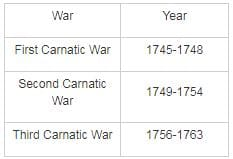RRB NTPC Mock Test - 13 (English) - Question 28

In each of the following questions find out the alternative which will replace the question mark.
PSMO : GMAE : : HSDB : ?

Detailed Solution for RRB NTPC Mock Test - 13 (English) - Question 28

PSMO and GMAE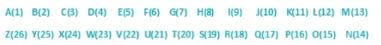Alphabetical position of P is 16. There are 16 letters in between P and G. (In reverse order)

Alphabetical position of S is 19. There are 19 letters in between S and M. (In reverse order)

Alphabetical position of M is 13. There are 13 letters in between M and A. (In reverse order)

Alphabetical position of O is 17. There are 17 letters in between O and E. (In reverse order)

Similary for HSDB

Alphabetical position of H is 8. There are 8 letters in between H and Q. (In reverse order)

Alphabetical position of S is 19. There are 19 letters in between S and M. (In reverse order)

Alphabetical position of D is 8. There are 8 letters in between D and Q. (In reverse order)

Alphabetical position of B is 2. There are 2 letters in between B and E. (In reverse order)

The word related to HSDB is QMIE.

RRB NTPC Mock Test - 13 (English) - Question 29

How many numbers less than 320 are multiples of both 5 and 3?

Detailed Solution for RRB NTPC Mock Test - 13 (English) - Question 29

Multiples of both 5 and 3 means multiples of 15

∴ Multiples of 15 that are less than 320 are,

⇒ 15, 30, 45, 60, 75, 90, 105, 120, 135, 150, 165, 180, 195, 210, 225, 240, 255, 270, 285, 300, 315

∴ There are 21 numbers less than 320 which are multiples of both 5 and 3

RRB NTPC Mock Test - 13 (English) - Question 30

Vilas remembers his marriage is after 4th July. While his sister remembers his marriage is before 6th July. On which date of July is his marriage?

Detailed Solution for RRB NTPC Mock Test - 13 (English) - Question 30

Vilas remembers his marriage is after 4th July (i.e. on 5th, 6th, 7th, etc.) while his sister remembers his marriage is before 6th July (i.e. on 5th, 4th, 3rd etc.). Combining both the statements we get only one possible date which is 5th July.

RRB NTPC Mock Test - 13 (English) - Question 31

The age of 4 people are 57, 34, 40 and 16. Which of the following cannot be the total age of any combination of these people if in the combination a person’s age can be used only once including minimum of 3 persons?

Detailed Solution for RRB NTPC Mock Test - 13 (English) - Question 31

The possible combinations are:

57 + 34 + 40 + 16 = 147

57 + 40 + 34 = 131

40 + 34 + 16 = 90

57 + 40 + 16 = 113

57 + 34 + 16 = 107

But combination for 114 is not possible.

Hence, 114 is the correct answer.

RRB NTPC Mock Test - 13 (English) - Question 32

A shopkeeper incurs 10% loss by selling a washing machine for Rs. 16200. At what price should the washing machine be sold to earn 15% profit?

Detailed Solution for RRB NTPC Mock Test - 13 (English) - Question 32

Let cost price of washing machine is x.

Cost price of washing machine when shopkeeper incurs 10% loss.

⇒ Cost price = [Selling price/(1 - loss)] × 100

⇒ Cost price = [16200/(1 - 10)] × 100 = 18000

Selling price of washing machine when shopkeeper earn 15% profit.

⇒ Selling price = Cost price × (1 + profit %)

⇒ Selling price = 18000 × (1 + 15/100)

⇒ Selling price = 18000 × 1.15 = Rs. 20700

RRB NTPC Mock Test - 13 (English) - Question 33

Direction: Study the following table carefully and answer the given question.

Table contains information about number of candidates applied for the post of Scientist in DRDO from various cities and respective passing percentage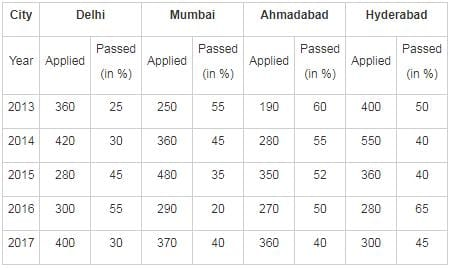Q. The total number of failed candidate from all cities together in year 2015 is?

Detailed Solution for RRB NTPC Mock Test - 13 (English) - Question 33

In year 2015,

Number of failed candidate from Delhi (A) = (100 - 45)% of 280 = 55% of 280 = 154

Number of failed candidate from Mumbai (B) = (100 - 35)% of 480 = 65% of 480 = 312

Number of failed candidate from Ahmadabad (C) = (100 - 52)% of 350 = 48% of 350 = 168

Number of failed candidate from Hyderabad (D) = (100 - 40)% of 360 = 60% of 360 = 216

∴ Total number of failed candidates = A + B + C + D = 154 + 312 + 168 + 216 = 850

RRB NTPC Mock Test - 13 (English) - Question 34

“Organ grade” body organization begins in animals from which phylum?

Detailed Solution for RRB NTPC Mock Test - 13 (English) - Question 34
• “Organ grade” body organization begins in animals from phylum Platyhelminthes.
• Before Platyhelminthes “tissue grade” of body organization is found in Phylum Coelenterata.
•  “Organ system grade” body organization starts from Phylum Aschelminthes.
• Phylum Annelida shows “metameric segmentation”.
RRB NTPC Mock Test - 13 (English) - Question 35

Select the related number from the given alternatives.
78 : 225 ∷ ? : 81

Detailed Solution for RRB NTPC Mock Test - 13 (English) - Question 35

As per the given information,

In 78 : 225 = 7 + 8 = 15 → 152 = 225

Similarly,

45 : 81 = 4 + 5 = 9 → 92 = 81

Hence, 45 is the correct answer.

RRB NTPC Mock Test - 13 (English) - Question 36

Who among the following is known as the Father of Indian Industry?

Detailed Solution for RRB NTPC Mock Test - 13 (English) - Question 36

● Jamsetji Tata is known as the father of Indian Industry.

● He was the founder of the Tata Group.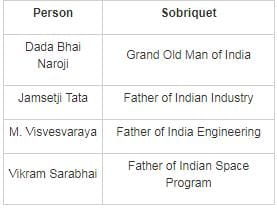RRB NTPC Mock Test - 13 (English) - Question 37

Average of 7 consecutive even numbers is 32. Which number is the largest of the 7 numbers?

Detailed Solution for RRB NTPC Mock Test - 13 (English) - Question 37

Let the 7 consecutive even numbers be x, (x + 2), (x + 4), (x + 6), (x + 8), (x + 10) and (x + 12).

Given,

Average of 7 consecutive even numbers = 32

⇒ Sum of 7 consecutive even numbers = 32 × 7 = 224

⇒ x + x + 2 + x + 4 + x + 6 + x + 8 + x + 10 + x + 12 = 224

⇒ 7x + 42 = 224

⇒ x = 182/7 = 26

∴ The largest of the 7 numbers = 26 + 12 = 38

RRB NTPC Mock Test - 13 (English) - Question 38

Who among the following built Kailashanatha temple?

Detailed Solution for RRB NTPC Mock Test - 13 (English) - Question 38

● Narasimhavarma II built the Kailashanatha temple.

● Kailashanatha temple is in Kanchi.

● It is dedicated to Lord Shiva and is known for its historical importance.

● Narasimhavarma II was the ruler of the Pallava Dynasty.

RRB NTPC Mock Test - 13 (English) - Question 39

Find out the correct answer for the unsolved equation based on a certain system

728 - 213 = 2, 549 - 123 = 2, 459 - 164 = ?

Detailed Solution for RRB NTPC Mock Test - 13 (English) - Question 39

The logic followed is:

728 - 213 = 2 → 72 ÷ 8 - 21 ÷ 3 = 9 - 7 = 2,

549 - 123 → 54 ÷ 9 - 12 ÷ 3 = 6 - 4 = 2,

Similarly,

459 - 164 → 45 ÷ 9 - 16 ÷ 4 = 5 - 4 = 1.

So, the missing term will be 1.

RRB NTPC Mock Test - 13 (English) - Question 40

During electro-refining, Pure Metal is collected at?

Detailed Solution for RRB NTPC Mock Test - 13 (English) - Question 40

● During electrorefining, Pure Metal is collected at Cathode.

● It is the process to remove impurities from a metal.

● The Pure metal is obtained and by the electrolysis method pure metal is plated on the cathode.

RRB NTPC Mock Test - 13 (English) - Question 41

Which among the following was the capital of Myanmar after getting Independence from British kingdom in 1948?

Detailed Solution for RRB NTPC Mock Test - 13 (English) - Question 41

● Yangon was the capital of Myanmar after getting Independence from British kingdom in 1948.

● It was also known as Rangoon.

● It is still the largest and one of the most important cities in Myanmar.

● Current capital: Naypyidaw

● Official currency: Kyat

RRB NTPC Mock Test - 13 (English) - Question 42

The average of 12 numbers is 6. If a number 10 is removed, then what will be the new average?

Detailed Solution for RRB NTPC Mock Test - 13 (English) - Question 42

Average of 12 numbers = 6

⇒ Sum of 12 numbers = 6 × 12 = 72

A number 10 is removed:

Then, the new sum = 72 - 10 = 62

∴ New Average = 62/11 = 5.63

RRB NTPC Mock Test - 13 (English) - Question 43

A seller offers 11% discount on a mini-refrigerator with a marked price of Rs. 8200. If he still earns a profit of Rs. 600, what is the cost price of the refrigerator?

Detailed Solution for RRB NTPC Mock Test - 13 (English) - Question 43

Let cost price of the mini-refrigerator = x

⇒ Marked price × (1 - discount%) = Selling price

⇒ 8200 × (1 - 11/100) = Selling price

⇒ Selling price = Rs. 7298

Cost price = Selling price - profit

⇒ x = 7298 - 600 = Rs. 6698

RRB NTPC Mock Test - 13 (English) - Question 44

Which among the following is the chemical name of caustic soda?

Detailed Solution for RRB NTPC Mock Test - 13 (English) - Question 44
• The chemical name of caustic soda Sodium Hydroxide.
• It is a white solid ionic compound.
• Formula: NaOH.
• It is soluble in Water, Ethanol, Methanol
RRB NTPC Mock Test - 13 (English) - Question 45

Which of the following country has won the 2019 South Asian Football Federation Women's Championship in Nepal?

Detailed Solution for RRB NTPC Mock Test - 13 (English) - Question 45

● India has won the South Asian Football Federation Women's Championship for the fifth time in a row.

● Indian team defeated hosts Nepal 3-1 in the final at Biratnagar in Nepal.

● This was India's 5th victory in the championship since its inception in 2010.

RRB NTPC Mock Test - 13 (English) - Question 46

In the following question, select the odd word pair from the given alternatives.

Detailed Solution for RRB NTPC Mock Test - 13 (English) - Question 46

All except 'Millet - Rabi' is the correct pair, Millet is a Kharif crop.

Hence, Millet - Rabi is the correct answer.

RRB NTPC Mock Test - 13 (English) - Question 47

A train is moving at the speed of 90 km/hr. how many seconds it will take to cover a distance of 2275 meter?

Detailed Solution for RRB NTPC Mock Test - 13 (English) - Question 47

Speed of train = Distance/Time

⇒ 90 × 5/18 = 2275/Time

∴ Time = 91 sec

RRB NTPC Mock Test - 13 (English) - Question 48

If x ∶ y = 3 ∶ 5, then what is the value of (2x + 5y) ∶ (2y - 3x)?

Detailed Solution for RRB NTPC Mock Test - 13 (English) - Question 48

Let x and y be 3a and 5a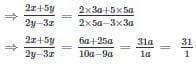RRB NTPC Mock Test - 13 (English) - Question 49

Select the related figure from the given alternatives: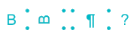Detailed Solution for RRB NTPC Mock Test - 13 (English) - Question 49

2nd image formed when 1st image is rotated at an angle of 90° in anticlockwise direction.

Hence, answer is option ‘3’.

RRB NTPC Mock Test - 13 (English) - Question 50

What is the difference (in Rs) between the compound interest (compounded annually) and simple interest for 3 years on a principal of Rs 2500 at the rate of 30% per annum?

Detailed Solution for RRB NTPC Mock Test - 13 (English) - Question 50

Given, Principal = Rs. 2500, Rate = 30%, Time = 3 years

When the interest is compounded annually,

Compound interest = Principal × [(1 + Rate/100)Time - 1]

⇒ Compound interest = 2500 × [(1 + 30/100)3 - 1] = 2500 × 1.197 = Rs. 2992.5

Simple interest = (Principal × Rate × Time)/100

⇒ Simple interest = (2500 × 30 × 3)/100 = Rs. 2250

∴ Required difference = 2992.5 - 2250 = Rs. 742.5

RRB NTPC Mock Test - 13 (English) - Question 51

In which of the following cities did the Great Smog occur in the year 1952?

Detailed Solution for RRB NTPC Mock Test - 13 (English) - Question 51
• The Great Smog of 1952 which is also known as the Killer Fog occurred in London.
• It took a standstill for four days i.e. 5 December, to, 9 December 1952 and it is estimated to have killed more than 4,000 people.
• Cold weather, combined with an anticyclone and windless conditions, collected airborne pollutants and formed a thick layer of smog.
• As visibility declined and the temperature dropped, people burned even more coal for heating and lighting, which resulted in ever-more polluted air.
RRB NTPC Mock Test - 13 (English) - Question 52

Correct the following equation by interchanging the two signs and two numbers.

25 ÷ 6 × 2 - 3 + 5 × 5 ÷ 5 = 77

Detailed Solution for RRB NTPC Mock Test - 13 (English) - Question 52

Given equation is 25 ÷ 6 × 2 - 3 + 5 × 5 ÷ 5 = 77

Let’s check each of the option,

1. Interchanging ×, ÷ and 5, - 3

Equation will become, 25 × 6 ÷ 2 + 5 - 3 ÷ 5 × 5 = 77

2. Interchanging +, ÷ and 2, 3

Equation will become, 25 + 6 × 3 - 2 ÷ 5 × 12 ÷ 3 = 41.4 ≠ 68

3. Interchanging ×, ÷ and 12, 3

Equation will become, 25 × 6 ÷ 2 - 3 + 5 × 3 ÷ 12 = 77.25 ≠ 68

4. Interchanging ×, - and 2, 3

Equation will become, 25 ÷ 6 - 3 × 2 + 5 × 12 ÷ 3 = 18 ≠ 68

Hence, by interchanging ×, ÷ and 5, - 3

We get correct answer.

RRB NTPC Mock Test - 13 (English) - Question 53

Choose the odd one out from the given alternatives.

Detailed Solution for RRB NTPC Mock Test - 13 (English) - Question 53

1) Damaged - Something which is broken/injured

2) Mistaken - An error

3) Wrong - Incorrect

4) Amiss - Faulty or wrong or mistaken

Hence, Damaged is odd word.

RRB NTPC Mock Test - 13 (English) - Question 54

Find the value of ‘x’ in the following question?
(2)4x + 3 × (2)3x – 5 = 4096

Detailed Solution for RRB NTPC Mock Test - 13 (English) - Question 54

Laws of Indices:

1. am × a= a{m + n}

2. a÷ a= a{m - n}

3. (am )= amn

4. (a)-m = 1/am

5. (a)m/n = n√am

6. (a)= 1

(2)4x + 3 × (2)3x – 5 = 4096

⇒ (2)4x + 3 × (2)3x – 5 = (2)12

⇒ (2){4x + 3 + 3x – 5} = (2)12

⇒ (2)7x – 2 = (2)12

⇒ 7x – 2 = 12

⇒ x = 14/7 = 2

∴ x = 2

RRB NTPC Mock Test - 13 (English) - Question 55

In which of the following year was WhatsApp started?

Detailed Solution for RRB NTPC Mock Test - 13 (English) - Question 55
• WhatsApp was started in2009.
• Its Headquarter is in California, United States.
• The founders of WhatsApp are Jan Koum& Brian Acton.
• It was acquired by Facebook in February 2014.
RRB NTPC Mock Test - 13 (English) - Question 56

A shopkeeper buys a fan for Rs 14500. For how much should he sell (in Rs) it to gain a profit of 10%?

Detailed Solution for RRB NTPC Mock Test - 13 (English) - Question 56

Cost price = C.P = Rs. 14500

profit % = (profit/C.P) × 100

⇒ 10 = (profit/14500) × 100

Profit = (10 × 14500)/100 = 1450

Profit = S.P - C.P

S.P = sell price

⇒ S.P = profit + C.P = 1450 + 14500 = Rs. 15950

Shortcut method:

Profit = 10%

Selling price = (1 + profit percent/100) × cost price

Selling price = (1 + 10/100) × 14500 = 1.1 × 14500 = Rs.15950

RRB NTPC Mock Test - 13 (English) - Question 57

Direction: A statement is given followed by two inferences I and II. You have to consider the statement to be true even if it seems to be at variance from commonly known facts. You have to decide which of the given inferences, if any, follow from the given statement.

Statement: Religions provide the means for attaining eternal peace.

Inference:
I. Religions ensure prosperous life.
II. Religions help people to eradicate poverty.​

Detailed Solution for RRB NTPC Mock Test - 13 (English) - Question 57

Option 4 is the correct answer. The statement says that religions are the way to achieve long-lasting peace which is not related to a prosperous or wealthy life. Also, it has no relation to poverty. Hence, both inferences are not followed.

RRB NTPC Mock Test - 13 (English) - Question 58

What is the value of cot 11π/6?

Detailed Solution for RRB NTPC Mock Test - 13 (English) - Question 58

⇒ cot(11π/6)

⇒ cot(2π – π/6)

⇒ -cot(π/6)
∵ cot is negative in fourth quadrant.

∴ - √3

RRB NTPC Mock Test - 13 (English) - Question 59

Who among the following presented the Interim Budget 2019-20 on February 1, 2019?

Detailed Solution for RRB NTPC Mock Test - 13 (English) - Question 59
• Interim Budget 2019-20 was presented by Piyush Goyal as Arun Jaitley undergoes medical treatment.

• The Indian Budget 2019 is of 27.84 lakh crore rupees.

• The expenditure has increased significantly when compared with the Union Budget 2018, where the expenditure figure was 24.42 lakh crore rupees.

• The total revenue expected in the financial year 2019-20 is Rs. 20.80 lakh crores.

RRB NTPC Mock Test - 13 (English) - Question 60

Elasticity expressed by formula 1 > e > 0 is ________.

Detailed Solution for RRB NTPC Mock Test - 13 (English) - Question 60

E = ∞ Perfectly elastic

E = 1 Unit elastic

E = 0 Perfectly inelastic

1 < E < ∞ Relatively elastic

1 > E > 0 Relatively inelastic

RRB NTPC Mock Test - 13 (English) - Question 61

Which among the following river does not originate in Indian Territory?

Detailed Solution for RRB NTPC Mock Test - 13 (English) - Question 61

● Sutlej does not originate in Indian Territory.

● It originates from Langqen Zangbo, China.

● It is also known as Satadree.

● It is the eastmost tributary of the Indus River.

RRB NTPC Mock Test - 13 (English) - Question 62

Which of the following device converts sound energy into electrical energy?

Detailed Solution for RRB NTPC Mock Test - 13 (English) - Question 62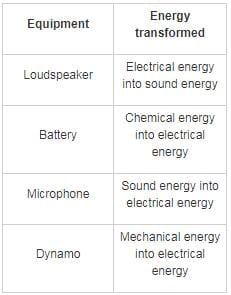RRB NTPC Mock Test - 13 (English) - Question 63

Which among the following has the highest thermal conductivity?

Detailed Solution for RRB NTPC Mock Test - 13 (English) - Question 63

● The thermal conductivity of a material is a measure of its ability to conduct heat.

●  The greater the value of thermal conductivity for a material, the more rapidly will it conduct heat.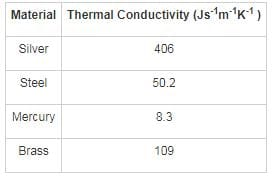RRB NTPC Mock Test - 13 (English) - Question 64

The ratio of the ages of Meena and Meera is 4 : 3 and the sum of their ages is 28 years. What will be the ratio of their ages after 8 years?

Detailed Solution for RRB NTPC Mock Test - 13 (English) - Question 64

Let the present age of Meena and Meera be 4x and 3x

Sum of ages = (4x + 3x) = 7x

7x = 28 ⇒ x = 4

8 years hence

Age of Meena = (4x + 8) = 24 years

Age of Meera = (3x + 8) = 20 years

Ratio of ages = 24/20 = 6 : 5

RRB NTPC Mock Test - 13 (English) - Question 65

How many rectangles are there in the given figure?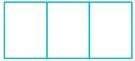Detailed Solution for RRB NTPC Mock Test - 13 (English) - Question 65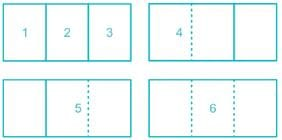Thus in the given figure there are 6 triangles.

RRB NTPC Mock Test - 13 (English) - Question 66

Which of the following is not an example of the indirect tax?

Detailed Solution for RRB NTPC Mock Test - 13 (English) - Question 66

● Income Tax is not an example of indirect tax, it comes under the direct tax.

● Indirect tax is a type of tax collected by the government from an intermediary and are not directly transferred to the government.

● Examples of Indirect tax are service tax, sales tax etc.

● A direct tax is borne directly by the entity that pays it.

● Examples of direct taxes are income tax, corporation tax etc.

RRB NTPC Mock Test - 13 (English) - Question 67

What is the chemical formula of Marsh Gas/Methane?​

Detailed Solution for RRB NTPC Mock Test - 13 (English) - Question 67
• The chemical formula of Marsh Gasis CH4.
• The chemical name of Marsh Gas is Methane.
• It is produced when vegetation decomposes naturally within some geographical marshes, swamps, and bogs.
• The main process for the production of methane is anaerobic digestion.
RRB NTPC Mock Test - 13 (English) - Question 68

Homeopathy was invented by ________.

Detailed Solution for RRB NTPC Mock Test - 13 (English) - Question 68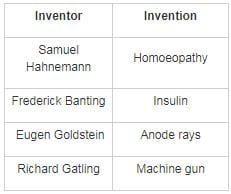RRB NTPC Mock Test - 13 (English) - Question 69

The speed of light was first measured by?

Detailed Solution for RRB NTPC Mock Test - 13 (English) - Question 69
• The speed of light was first measured by Olaus Roemer.
• Speed of light = 3 ×108 m/s
• Light is a form of energy which is propagated as electromagnetic waves.
• The speed of light is maximum in Vacuum followed Air.
RRB NTPC Mock Test - 13 (English) - Question 70

Neuschwanstein Castle is located in which of the following country?

Detailed Solution for RRB NTPC Mock Test - 13 (English) - Question 70

● Neuschwanstein Castle is located in southwest Bavaria, Germany.

● It was commissioned by Ludwig II of Bavaria as a retreat and in honour of Richard Wagner.

● It was opened for the public in 1886.

RRB NTPC Mock Test - 13 (English) - Question 71

Computer programming language FORTRAN is used to

I. Numeric computation
II. Create web pages
III. Draw objects

Detailed Solution for RRB NTPC Mock Test - 13 (English) - Question 71

● FORTRAN is a programming language that is used for numeric computation and scientific computing.

● It stands for FORmula TRANslation.

● It shortened the process of programming and made computer programming more accessible.

● It was developed by a team of programmers at IBM led by John Backus.

RRB NTPC Mock Test - 13 (English) - Question 72

What is the sound made by bats called?

Detailed Solution for RRB NTPC Mock Test - 13 (English) - Question 72
• The sound made by bats is known as a Screech.

Sounds made by other animals are as follow:

• Antelope - Snort
• Badgers - Growl
• Chimpanzees – Scream
RRB NTPC Mock Test - 13 (English) - Question 73

Which figure represents the relation among Lentils, Wheat and Maize?

Detailed Solution for RRB NTPC Mock Test - 13 (English) - Question 73

There is no relation between lentils, Wheat and Maize.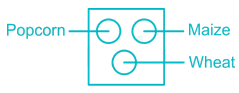Hence, the correct answer is option 3.

RRB NTPC Mock Test - 13 (English) - Question 74

Which of the following body part is affected by the disease Rhinitis?

Detailed Solution for RRB NTPC Mock Test - 13 (English) - Question 74
• Rhinitis affects the nose.
• It causes irritation and inflammation in the mucous membrane present in the nose.
• It is also known as coryza.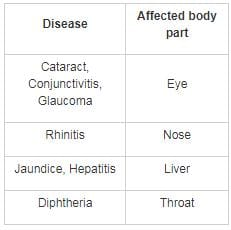RRB NTPC Mock Test - 13 (English) - Question 75

An article is sold at a loss of 20%. If the selling price is doubled, then what will be the profit percentage?

Detailed Solution for RRB NTPC Mock Test - 13 (English) - Question 75

Let the cost price of article be x.

Loss % = 20%

Then, SP = CP - Loss

Loss = 20% of x = 0.2x

⇒ SP = x - 0.2x = 0.8x

If the selling price is doubled,

SP = 2 × 0.8x = 1.6x

Profit = SP - CP = 1.6x - x = 0.6x

Profit % = (Profit × 100)/CP

Profit % = (0.6x × 100)/x = 60%

RRB NTPC Mock Test - 13 (English) - Question 76

6 members of a family works in a company. S’s son is Z. B is married to S. Y and S are siblings. D is brother of Z. F is daughter of B. Y is a male. Mother of Z is not S. How is D related to Y?

Detailed Solution for RRB NTPC Mock Test - 13 (English) - Question 76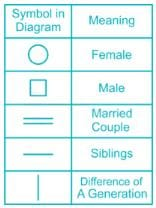According to the given information, we get the following tree diagram,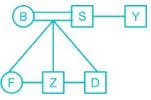From the above tree, we can conclude that D is nephew of Y.

RRB NTPC Mock Test - 13 (English) - Question 77

Which of the following country will host the 2022 FIFA World Cup?

Detailed Solution for RRB NTPC Mock Test - 13 (English) - Question 77

● The 2022 FIFA World Cup will be held at Qatar from 21 November - 18 December 2022.

● This will be the first FIFA world cup that will be hosted in a Muslim majority country and also in an Arab country.

● The 2018 FIFA World Cup took place in Russia from 14 June to 15 July 2018.

● France won the 2018 FIFA World Cup by defeating Croatia in the finals.

RRB NTPC Mock Test - 13 (English) - Question 78

Arrange the given words in the sequence in which they occur in the dictionary.

i. Mouse
ii. Manage
iii. Magic
iv. Machine

Detailed Solution for RRB NTPC Mock Test - 13 (English) - Question 78

On arranging given word as per dictionary order,

iv Machine

iiiMagic

iiManage

Mouse

Hence, “iv, iii, ii, i” is correct order as per the dictionary.

RRB NTPC Mock Test - 13 (English) - Question 79

Gregor Mendel had used which of this plants to cross breed for his experiment?

Detailed Solution for RRB NTPC Mock Test - 13 (English) - Question 79
• Gregor Mendel is known as the father of genetics. He conducted a pea plant experiment during 1856-63 and established several rules of heredity, which are now called the laws of Mendelian Inheritance.
• The terms dominant and recessive were also coined by him during this experiment.
RRB NTPC Mock Test - 13 (English) - Question 80

HDFC in HDFC bank stands for ________.

Detailed Solution for RRB NTPC Mock Test - 13 (English) - Question 80

● HDFC stands for Housing Development Finance Corporation.

● It is India’s largest private sector lender by assets.

● Founded: August 1994

● Managing Director: Aditya Puri

RRB NTPC Mock Test - 13 (English) - Question 81

The co-ordinates of the centroid of a triangle ABC are (2, 2). What are the co-ordinates of vertex C if co-ordinates of A and B are (7, -1) and (1, 2) respectively?

Detailed Solution for RRB NTPC Mock Test - 13 (English) - Question 81

Let the coordinate of C be (x, y)

Centroid of triangle can be given as

For X coordinate,

⇒ 2 = (x + 7 + 1)/3

⇒ x = -2

For Y coordinate,

⇒ 2 = (y - 1 + 2)/3

⇒ y = 5

∴ The coordinate of C is (-2, 5)

RRB NTPC Mock Test - 13 (English) - Question 82

If ‘A’ denotes ‘÷’, ‘B’ denotes ‘×’, ‘C’ denotes ‘+’, ‘D’ denotes ‘- ‘, then find the value of
68 C ((30 D 200) B 10) A 2?

Detailed Solution for RRB NTPC Mock Test - 13 (English) - Question 82Given: 68 C ((30 D 200) B 10) A 2

Substituting the symbols:

68 + ((30 - 200) × 10) ÷ 2

Solution through BODMAS:

68 + ((30 - 200) × 10) ÷ 2

68 + (( - 1700) ÷ 2 = - 782

Hence, the correct answer is - 782.

RRB NTPC Mock Test - 13 (English) - Question 83

A series is given with one term missing. Select the correct alternative from the given ones that will complete the series.
NET, OGW, PIZ, QKC, ?

Detailed Solution for RRB NTPC Mock Test - 13 (English) - Question 83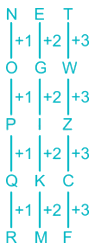Hence, the correct answer is RMF.

RRB NTPC Mock Test - 13 (English) - Question 84

In certain language, ‘pan’ tan san' means 'pen is gold’, 'tan san van' means 'gold is old', 'san van kan' means ‘old is bold’, 'kan san haw' means ‘bold is strong’. How is 'strong is pen' coded in that language?

Detailed Solution for RRB NTPC Mock Test - 13 (English) - Question 84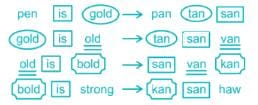'pan' → pen

'san' → is

'haw' → strong

So, 'strong is pen' is coded as ' pan haw san'.

Therefore, ‘pan haw san' is the correct answer.

RRB NTPC Mock Test - 13 (English) - Question 85

Read the given question and decide which of the following argument is/are strong.
Should all indirect taxes in India be combined into a single tax on all commodities?

Arguments:
1. Yes, this will considerably simplify the tax collection mechanism and the cost of collection of taxes will also reduce.
2. No, no other country has adopted such system.

Detailed Solution for RRB NTPC Mock Test - 13 (English) - Question 85

Only 1st argument is strong as it provides very convincing information in favour of a single tax system for commodities. The 2nd argument does not hold strong as it does not provide enough information. No other country adopting this system of taxation does not mean that it is not a profitable venture.

RRB NTPC Mock Test - 13 (English) - Question 86

The floral part that produces Pollen Grain is _________.

Detailed Solution for RRB NTPC Mock Test - 13 (English) - Question 86

● The floral part that produces Pollen Grain is Anther.

● Stamens are the structure that produces pollen terminal sac-like structure called Anthers.

● Petals surround the carpel and stamen and are brightly coloured.

● Sepals surround the flower petals before they open.

● Below the style is an enlarged area called the ovary. The ovary is where a new plant begins to form.

RRB NTPC Mock Test - 13 (English) - Question 87

In certain code ‘QUITE’ is written as ‘5\$?1@’ and ‘TINY’ is written as ‘1?6#’. How is ‘NET’ written in that code?

Detailed Solution for RRB NTPC Mock Test - 13 (English) - Question 87

Assigning codes to respective letters: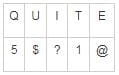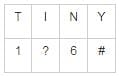Similarly,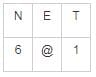Hence, the code for NET is 6@1.

RRB NTPC Mock Test - 13 (English) - Question 88

If the variance of a distribution is 81 and the coefficient variation is 30%, find mean.

Detailed Solution for RRB NTPC Mock Test - 13 (English) - Question 88

We know,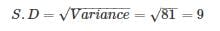And,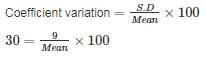⇒ Mean = 30

RRB NTPC Mock Test - 13 (English) - Question 89

What will come in place of question mark (?) in the following question?
84% of 2500 – 420 = 350% of ?

Detailed Solution for RRB NTPC Mock Test - 13 (English) - Question 89

Given expression is,
84% of 2500 – 420 = 350% of ?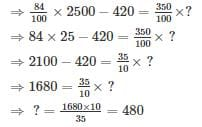∴ ? = 480

RRB NTPC Mock Test - 13 (English) - Question 90

A series is given with one term missing. Select the correct alternative from the given ones that will complete the series.
0, 9, 17, 24, 30, 35, ? , 42

Detailed Solution for RRB NTPC Mock Test - 13 (English) - Question 90

The given series is:

(0 + 10) – 1 = 9

(9 + 9) – 1 = 17

(17 + 8) – 1 = 24

(24 + 7) – 1 = 30

(30 + 6) – 1 = 35

(35 + 5) – 1 = 39

(39 + 4) – 1 = 42

Hence, the required number is 39.

RRB NTPC Mock Test - 13 (English) - Question 91

If a mirror is placed on the line AB, then which of the answer figures is the right image of the given figure?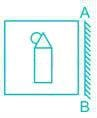Detailed Solution for RRB NTPC Mock Test - 13 (English) - Question 91

The mirror image of the question will be: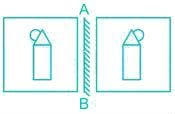Hence, we can say that option 3 is the correct mirror image of the given question.

RRB NTPC Mock Test - 13 (English) - Question 92

Which of the following planet is not a terrestrial planet?

Detailed Solution for RRB NTPC Mock Test - 13 (English) - Question 92
• The planets that are composed of silicate rocks or metals are termed as terrestrial planets.
• The inner planets closest to the Sun are terrestrial planets i.e. Mercury, Venus, Earth, and Mars.
• They have a central metallic core, mostly iron, with a surrounding silicate mantle.
• Only one terrestrial planet, Earth, is known to have an active hydrosphere.
RRB NTPC Mock Test - 13 (English) - Question 93

What is the value equation a3 + b3 + c3 - 3abc if a2 + b2 + c2 = ab + bc + ca + 4 and a + b + c = 4.

Detailed Solution for RRB NTPC Mock Test - 13 (English) - Question 93

As we know, (a3 + b3 + c3 – 3abc) = (a + b + c)(a2 + b2 + c2 – ab – bc – ca)

Given, a2 + b2 + c2 = ab + bc + ca + 4

⇒ a2 + b2 + c2 – ab – bc – ca = 4

∴ (a3 + b3 + c3 – 3abc) = 4 × 4 = 16

RRB NTPC Mock Test - 13 (English) - Question 94

When was the Indian Citizenship Act passed?

Detailed Solution for RRB NTPC Mock Test - 13 (English) - Question 94
• Indian Citizenship Act was passed in 1955.
• As per Citizenship Act, 1955 one can lose the citizenship of India in three ways: Renunciation, Termination and Deprivation.

Indian Citizenship can be acquired under the following ways

• Citizenship by birth
• Citizenship by descent
• Citizenship by registration
• Citizenship by naturalization
• Citizenship by incorporation of a territory
RRB NTPC Mock Test - 13 (English) - Question 95

If (9 - 3x) - (17x - 10) = - 1, then the value of x is

Detailed Solution for RRB NTPC Mock Test - 13 (English) - Question 95

(9 - 3x) - (17x - 10) = - 1

⇒ 9 - 3x - 17x + 10 = - 1

⇒ 20x = 20

⇒ x = 1

RRB NTPC Mock Test - 13 (English) - Question 96

Directions: In the question below is given a statement followed by two conclusions numbered I and II. You have to assume everything in the statement to be true, then consider the two conclusions together and decide which of them logically followed beyond a reasonable doubt from the information given in the statement. Give answer.

Statement: Every politician cannot be a political scientist as not all of the former possess the required qualification.

Conclusions:
I. Politicians necessarily need to be illiterate.
II. A political scientists will necessarily make a good politician.

Detailed Solution for RRB NTPC Mock Test - 13 (English) - Question 96

From the given statement it can be concluded that it is not necessary that all politicians are as educated to be called a political scientist. However, this does not imply that all the politicians have to be illiterate. Thus I does not follow. II cannot be concluded from the given statement as compares the politicians to the political scientists and not the other way round. Also, it is not necessary that a well educated person makes a good politician.

RRB NTPC Mock Test - 13 (English) - Question 97

What will come in place of question mark ‘?’ in the following question?
13 × (22 + 3) - 3 × (15 - 5)2 - 15 = ? × 5

Detailed Solution for RRB NTPC Mock Test - 13 (English) - Question 97

Follow BODMAS rule to solve this question, as per the order given below.

Step - 1 - steps of an equation enclosed in ‘Brackets’ must be solved first.

Step - 2 - any mathematical ‘Of’ or ‘Exponent’ must be solved next.

Step - 3 - Next, the parts of the equation that contain ‘Division’ and ‘Multiplication’ are calculated.

Step - 4 - Last but not least, the parts of the equation that contain ‘Addition’ and ‘Subtraction’ should be calculated.

Now, the given expression:

⇒ 13 × (22 + 3) - 3 × (15 - 5)2 - 15 = ? × 5

⇒ 13 × 25 - 3 × (10)2 - 15 = ? × 5

⇒ 13 × 25 - 3 × 100 - 15 = ? × 5

⇒ 325 - 300 - 15 = ? × 5

⇒ ? × 5 = 25 - 15

⇒ ? = 10/5

⇒ ? = 2

RRB NTPC Mock Test - 13 (English) - Question 98

In which of the following year, the Chaura Chauri incident took place?

Detailed Solution for RRB NTPC Mock Test - 13 (English) - Question 98
• The Chaura Chauri incident took place on February 5, 1922, in the Gorakhpur district of British India.
• In this incident, the crowd that was participating in the Non-Cooperation movement clashed with police and the incident turned violent.
• In the result, the mob attacked and set fire to a police station.
• It resulted in the death of 22 or 23 policemen and three civilians.
RRB NTPC Mock Test - 13 (English) - Question 99

Direction: Read the following information carefully and answer the questions that follow:
Five boys M, N, O, P and Q are sitting around a circular table facing the centre. O sits third to the right of M. P is not the neighbor of M. Q is not the neighbor of O.

Q. Who is third to the left of the person who is adjacent to N?

Detailed Solution for RRB NTPC Mock Test - 13 (English) - Question 99

As per the given instructions:

1) O sits third to the right of M.

2) P is not the neighbor of M.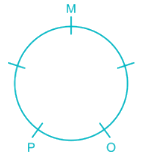3) Q is not the neighbor of O.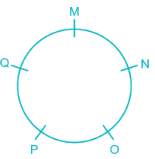Adjacent to N are M and O:

Third to the left of M is P and third to the left of O is M.

RRB NTPC Mock Test - 13 (English) - Question 100

P, Q, R, S, T, U, V and W are sitting round the circle and are facing the centre:
P is second to the right of T who is the neighbour of R and V.
S is not the neighbour of P.
V is the neighbour of U.
Q is not between S and W.
W is not between U and S.
Q. Which two of the following are not neighbours ?

Detailed Solution for RRB NTPC Mock Test - 13 (English) - Question 100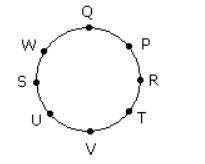## RRB NTPC Mock Tests Series

20 tests
 Use Code STAYHOME200 and get INR 200 additional OFF Use Coupon Code
Information about RRB NTPC Mock Test - 13 (English) Page
In this test you can find the Exam questions for RRB NTPC Mock Test - 13 (English) solved & explained in the simplest way possible. Besides giving Questions and answers for RRB NTPC Mock Test - 13 (English), EduRev gives you an ample number of Online tests for practice

20 tests